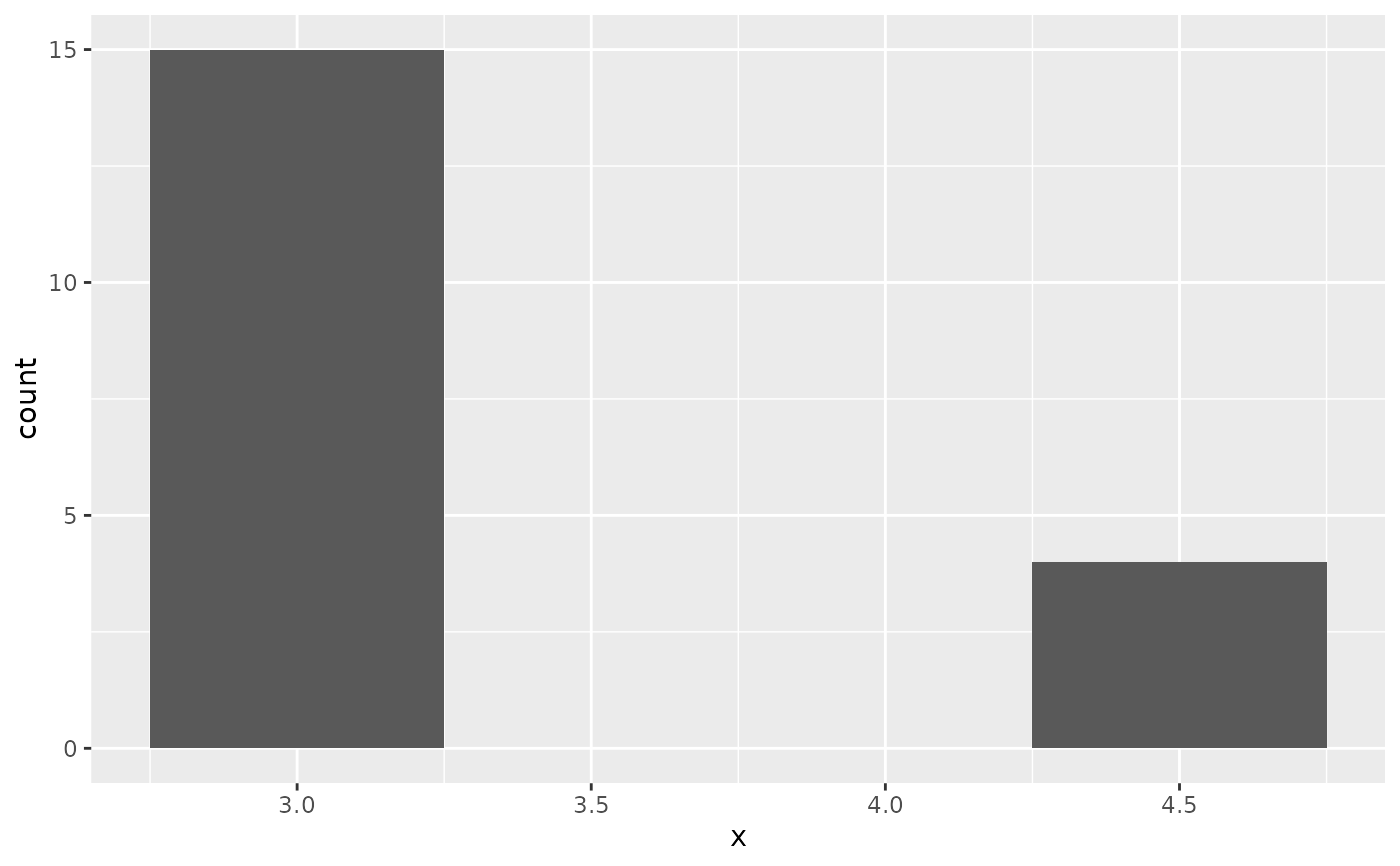There are two types of bar charts: geom_bar() and geom_col(). geom_bar() makes the height of the bar proportional to the number of cases in each group (or if the weight aesthetic is supplied, the sum of the weights). If you want the heights of the bars to represent values in the data, use geom_col() instead. geom_bar() uses stat_count() by default: it counts the number of cases at each x position. geom_col() uses stat_identity(): it leaves the data as is.

## Usage

geom_bar(
mapping = NULL,
data = NULL,
stat = "count",
position = "stack",
...,
width = NULL,
na.rm = FALSE,
orientation = NA,
show.legend = NA,
inherit.aes = TRUE
)

geom_col(
mapping = NULL,
data = NULL,
position = "stack",
...,
width = NULL,
na.rm = FALSE,
show.legend = NA,
inherit.aes = TRUE
)

stat_count(
mapping = NULL,
data = NULL,
geom = "bar",
position = "stack",
...,
width = NULL,
na.rm = FALSE,
orientation = NA,
show.legend = NA,
inherit.aes = TRUE
)

## Arguments

mapping

Set of aesthetic mappings created by aes() or aes_(). If specified and inherit.aes = TRUE (the default), it is combined with the default mapping at the top level of the plot. You must supply mapping if there is no plot mapping.

data

The data to be displayed in this layer. There are three options:

If NULL, the default, the data is inherited from the plot data as specified in the call to ggplot().

A data.frame, or other object, will override the plot data. All objects will be fortified to produce a data frame. See fortify() for which variables will be created.

A function will be called with a single argument, the plot data. The return value must be a data.frame, and will be used as the layer data. A function can be created from a formula (e.g. ~ head(.x, 10)).

position

Position adjustment, either as a string, or the result of a call to a position adjustment function.

...

Other arguments passed on to layer(). These are often aesthetics, used to set an aesthetic to a fixed value, like colour = "red" or size = 3. They may also be parameters to the paired geom/stat.

width

Bar width. By default, set to 90% of the resolution of the data.

na.rm

If FALSE, the default, missing values are removed with a warning. If TRUE, missing values are silently removed.

orientation

The orientation of the layer. The default (NA) automatically determines the orientation from the aesthetic mapping. In the rare event that this fails it can be given explicitly by setting orientation to either "x" or "y". See the Orientation section for more detail.

show.legend

logical. Should this layer be included in the legends? NA, the default, includes if any aesthetics are mapped. FALSE never includes, and TRUE always includes. It can also be a named logical vector to finely select the aesthetics to display.

inherit.aes

If FALSE, overrides the default aesthetics, rather than combining with them. This is most useful for helper functions that define both data and aesthetics and shouldn't inherit behaviour from the default plot specification, e.g. borders().

geom, stat

Override the default connection between geom_bar() and stat_count().

## Details

A bar chart uses height to represent a value, and so the base of the bar must always be shown to produce a valid visual comparison. Proceed with caution when using transformed scales with a bar chart. It's important to always use a meaningful reference point for the base of the bar. For example, for log transformations the reference point is 1. In fact, when using a log scale, geom_bar() automatically places the base of the bar at 1. Furthermore, never use stacked bars with a transformed scale, because scaling happens before stacking. As a consequence, the height of bars will be wrong when stacking occurs with a transformed scale.

By default, multiple bars occupying the same x position will be stacked atop one another by position_stack(). If you want them to be dodged side-to-side, use position_dodge() or position_dodge2(). Finally, position_fill() shows relative proportions at each x by stacking the bars and then standardising each bar to have the same height.

## Orientation

This geom treats each axis differently and, thus, can thus have two orientations. Often the orientation is easy to deduce from a combination of the given mappings and the types of positional scales in use. Thus, ggplot2 will by default try to guess which orientation the layer should have. Under rare circumstances, the orientation is ambiguous and guessing may fail. In that case the orientation can be specified directly using the orientation parameter, which can be either "x" or "y". The value gives the axis that the geom should run along, "x" being the default orientation you would expect for the geom.

## Aesthetics

geom_bar() understands the following aesthetics (required aesthetics are in bold):

• x

• y

• alpha

• colour

• fill

• group

• linetype

• size

Learn more about setting these aesthetics in vignette("ggplot2-specs").

geom_col() understands the following aesthetics (required aesthetics are in bold):

• x

• y

• alpha

• colour

• fill

• group

• linetype

• size

Learn more about setting these aesthetics in vignette("ggplot2-specs").

stat_count() understands the following aesthetics (required aesthetics are in bold):

• x or y

• group

• weight

Learn more about setting these aesthetics in vignette("ggplot2-specs").

## Computed variables

count

number of points in bin

prop

groupwise proportion

geom_histogram() for continuous data, position_dodge() and position_dodge2() for creating side-by-side bar charts.

stat_bin(), which bins data in ranges and counts the cases in each range. It differs from stat_count(), which counts the number of cases at each x position (without binning into ranges). stat_bin() requires continuous x data, whereas stat_count() can be used for both discrete and continuous x data.

## Examples

# geom_bar is designed to make it easy to create bar charts that show
# counts (or sums of weights)
g <- ggplot(mpg, aes(class))
# Number of cars in each class:
g + geom_bar()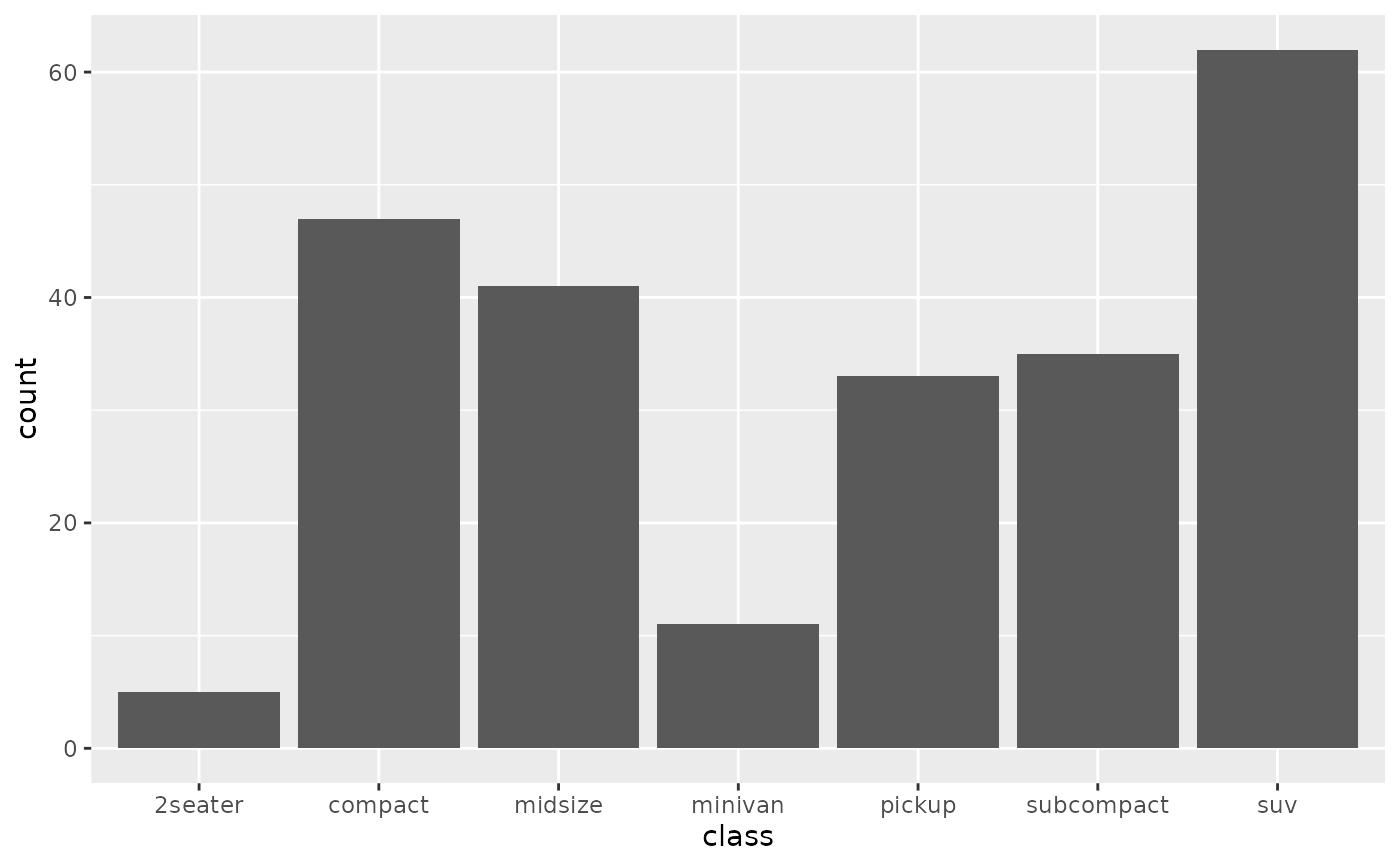# Total engine displacement of each class
g + geom_bar(aes(weight = displ))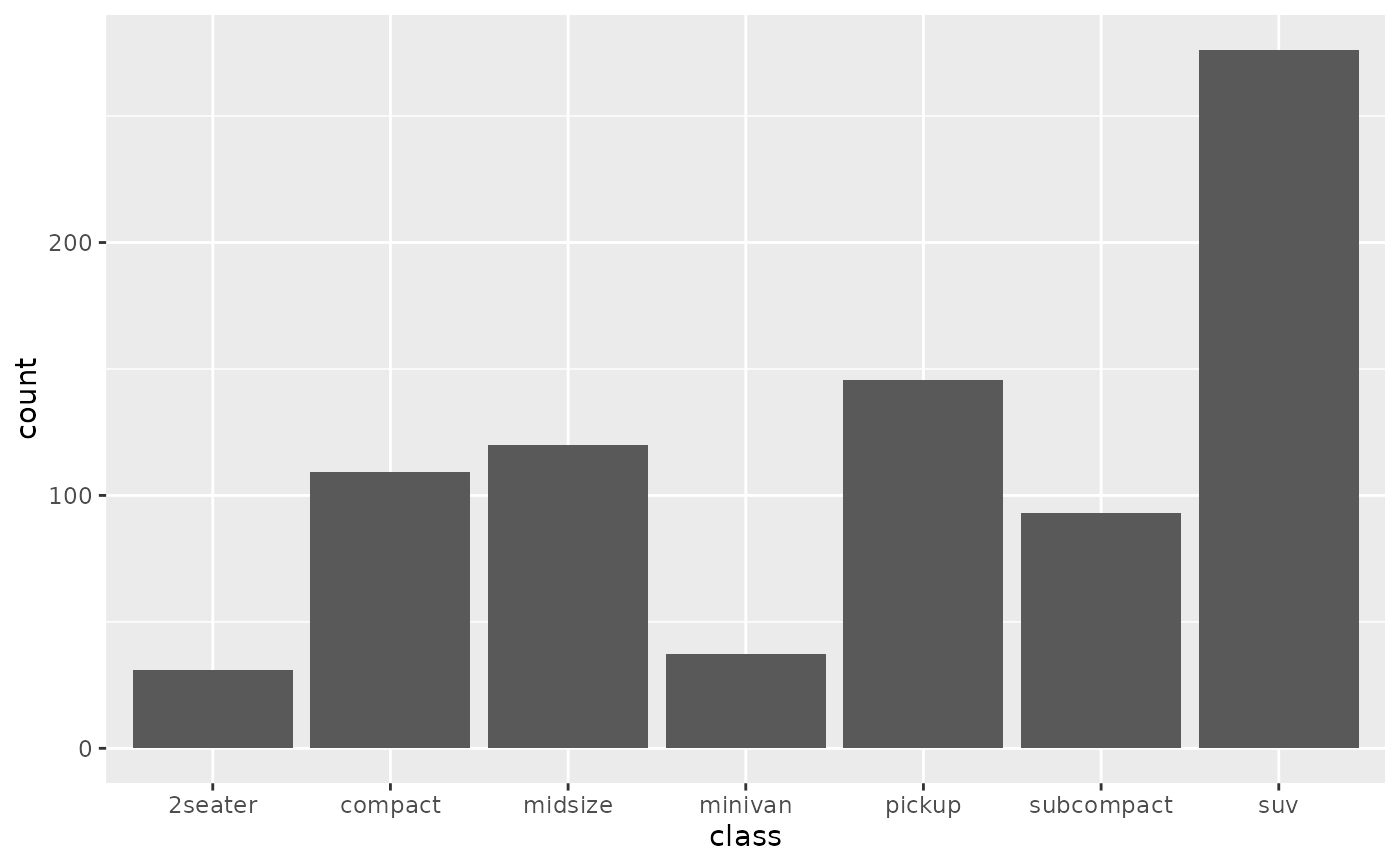# Map class to y instead to flip the orientation
ggplot(mpg) + geom_bar(aes(y = class))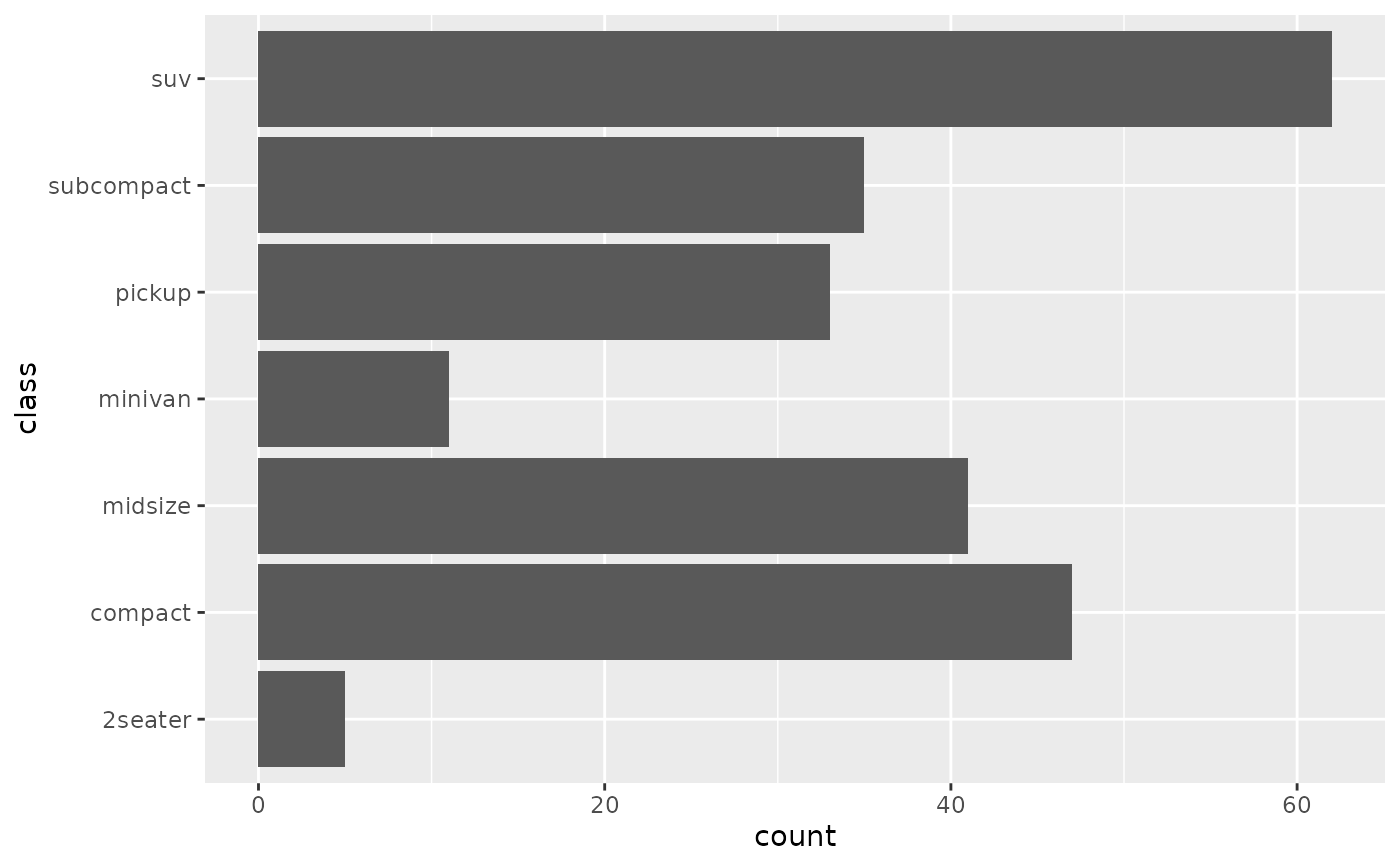# Bar charts are automatically stacked when multiple bars are placed
# at the same location. The order of the fill is designed to match
# the legend
g + geom_bar(aes(fill = drv))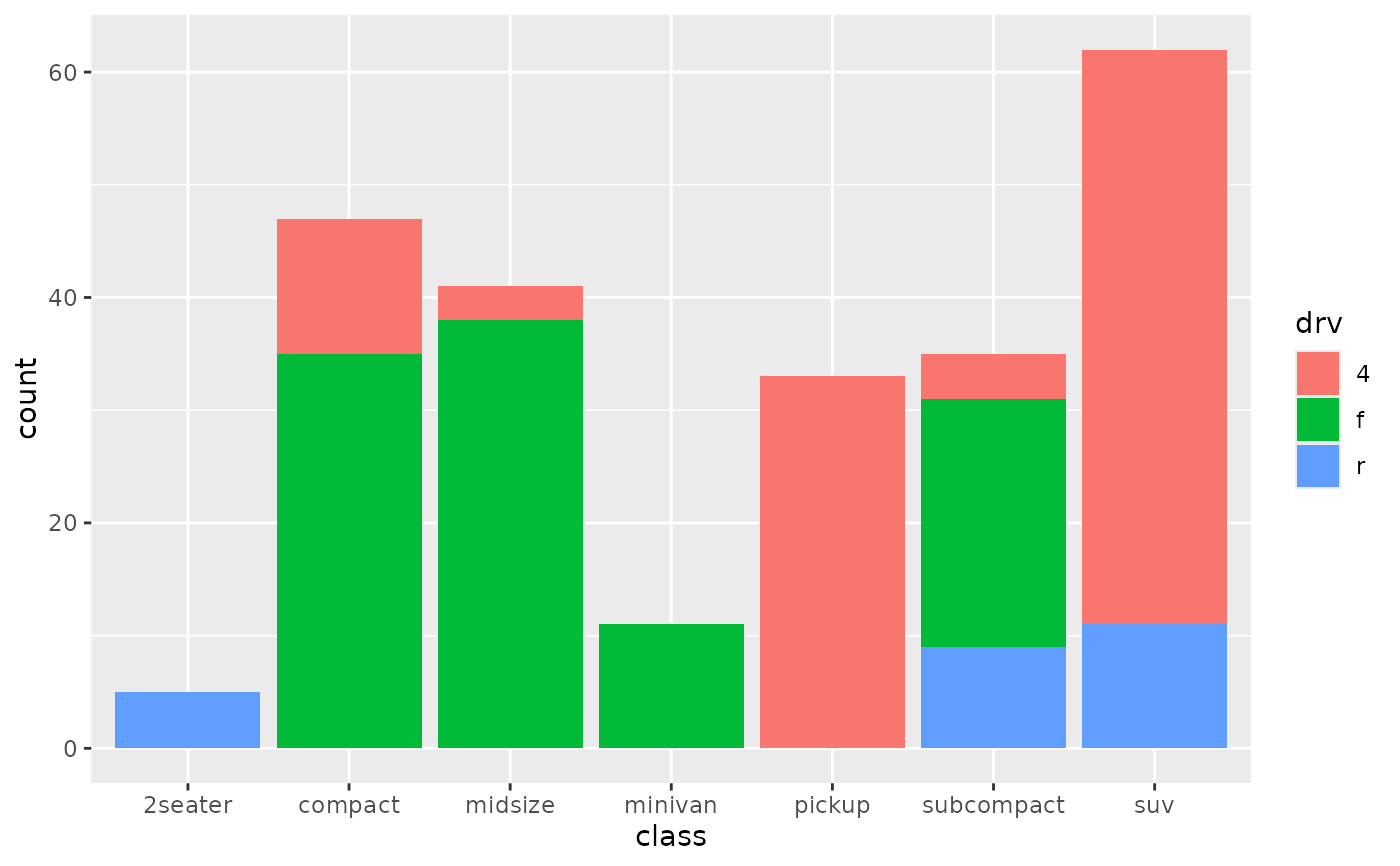# If you need to flip the order (because you've flipped the orientation)
# call position_stack() explicitly:
ggplot(mpg, aes(y = class)) +
geom_bar(aes(fill = drv), position = position_stack(reverse = TRUE)) +
theme(legend.position = "top")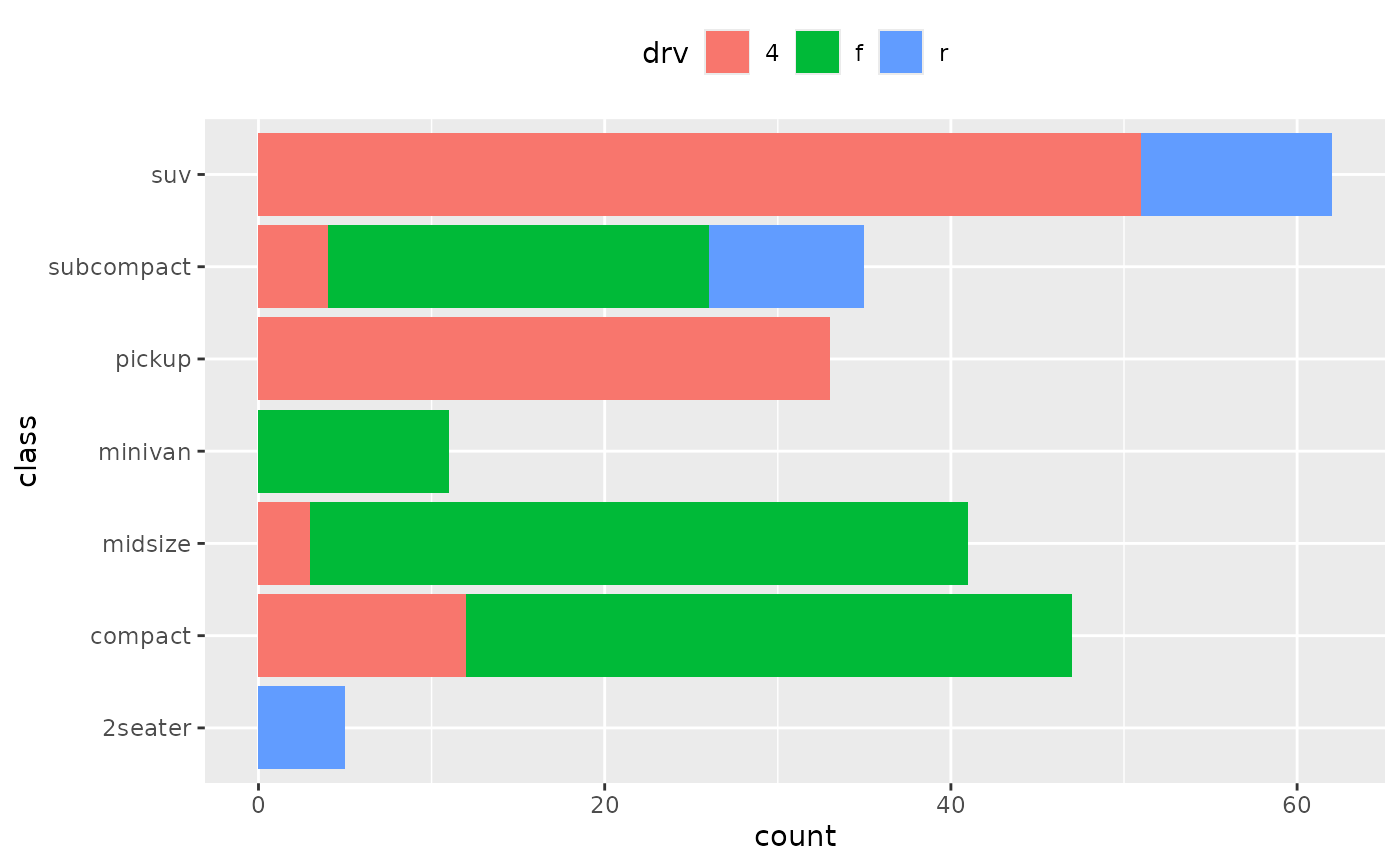# To show (e.g.) means, you need geom_col()
df <- data.frame(trt = c("a", "b", "c"), outcome = c(2.3, 1.9, 3.2))
ggplot(df, aes(trt, outcome)) +
geom_col()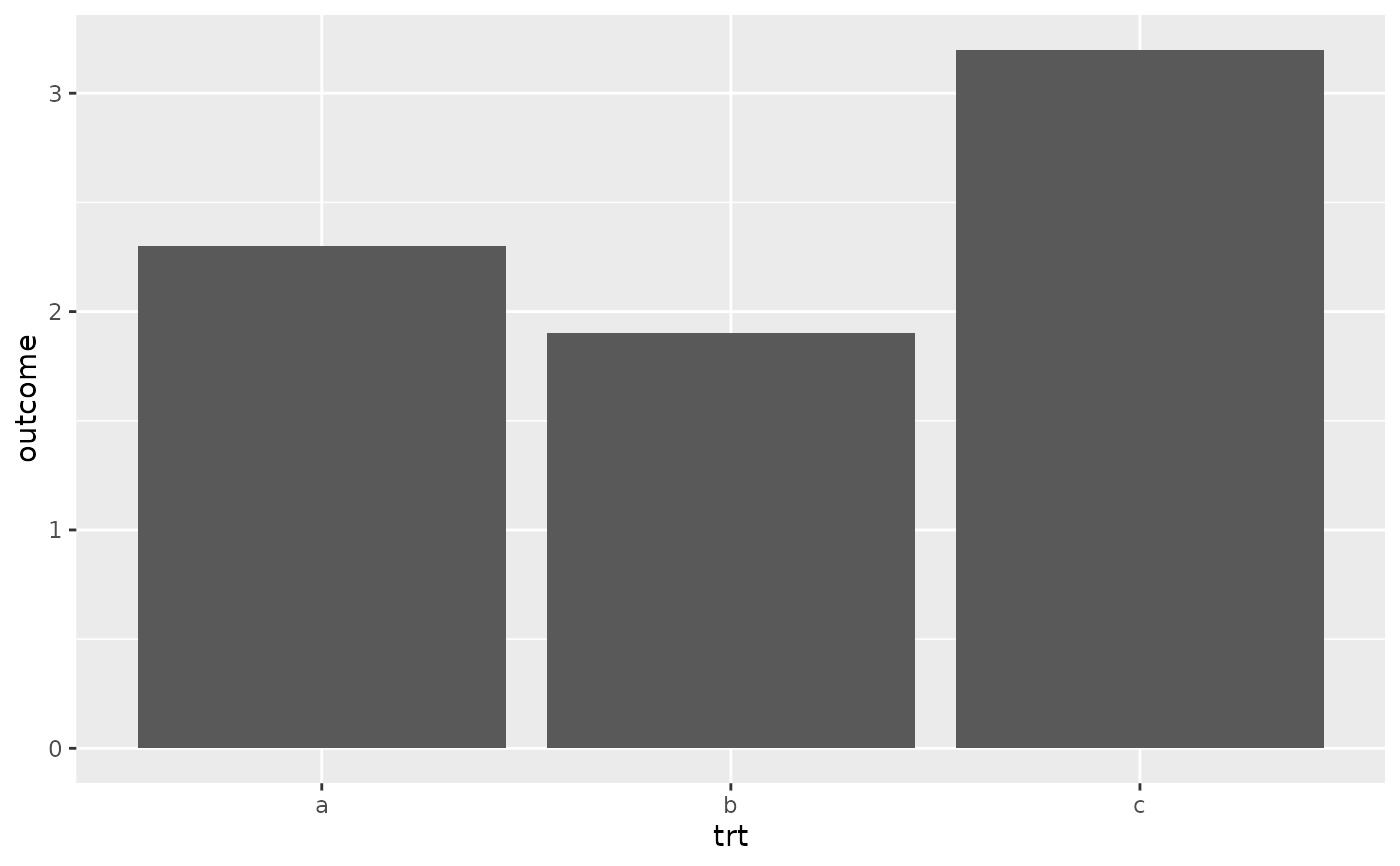# But geom_point() displays exactly the same information and doesn't
# require the y-axis to touch zero.
ggplot(df, aes(trt, outcome)) +
geom_point()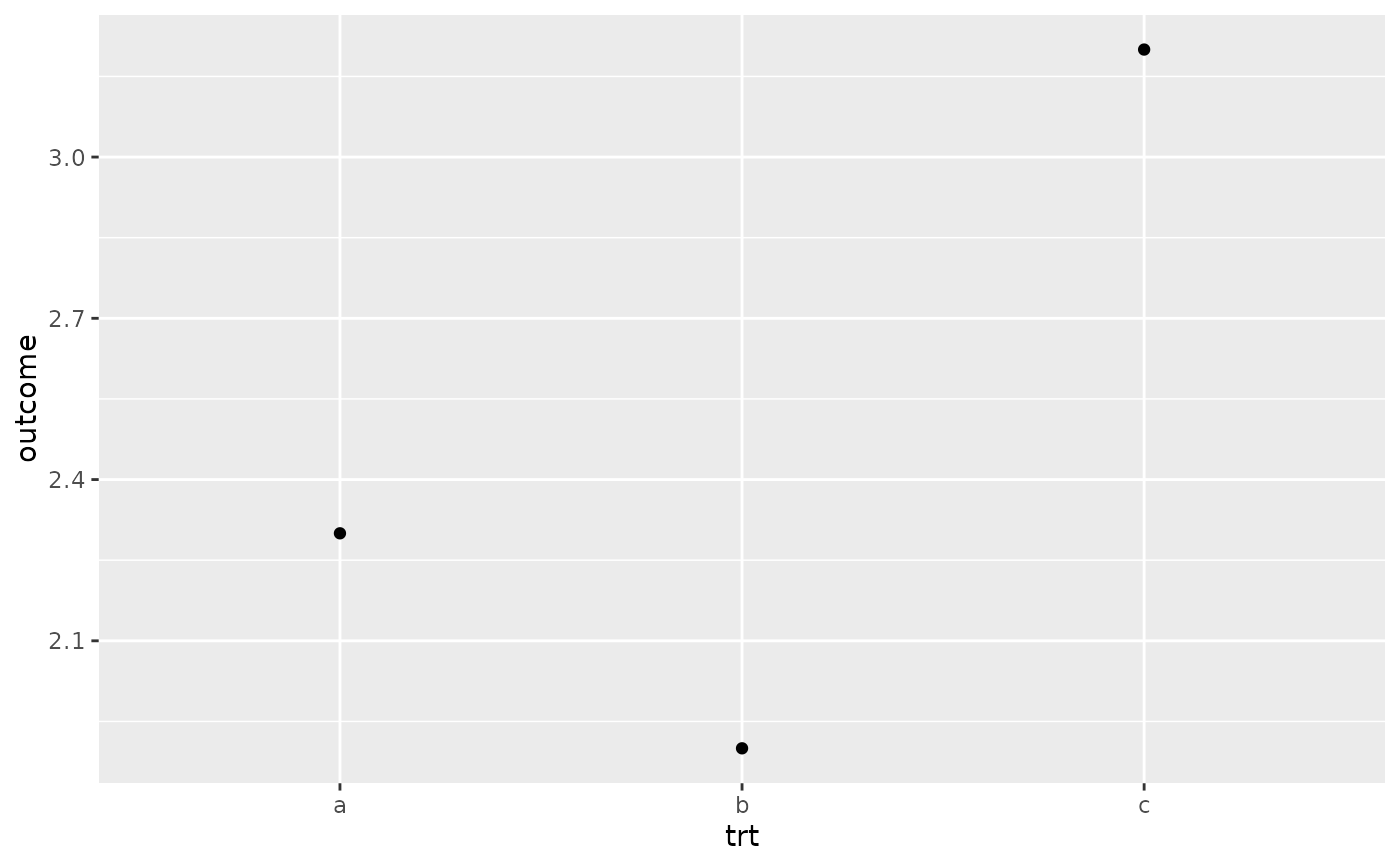# You can also use geom_bar() with continuous data, in which case
# it will show counts at unique locations
df <- data.frame(x = rep(c(2.9, 3.1, 4.5), c(5, 10, 4)))
ggplot(df, aes(x)) + geom_bar()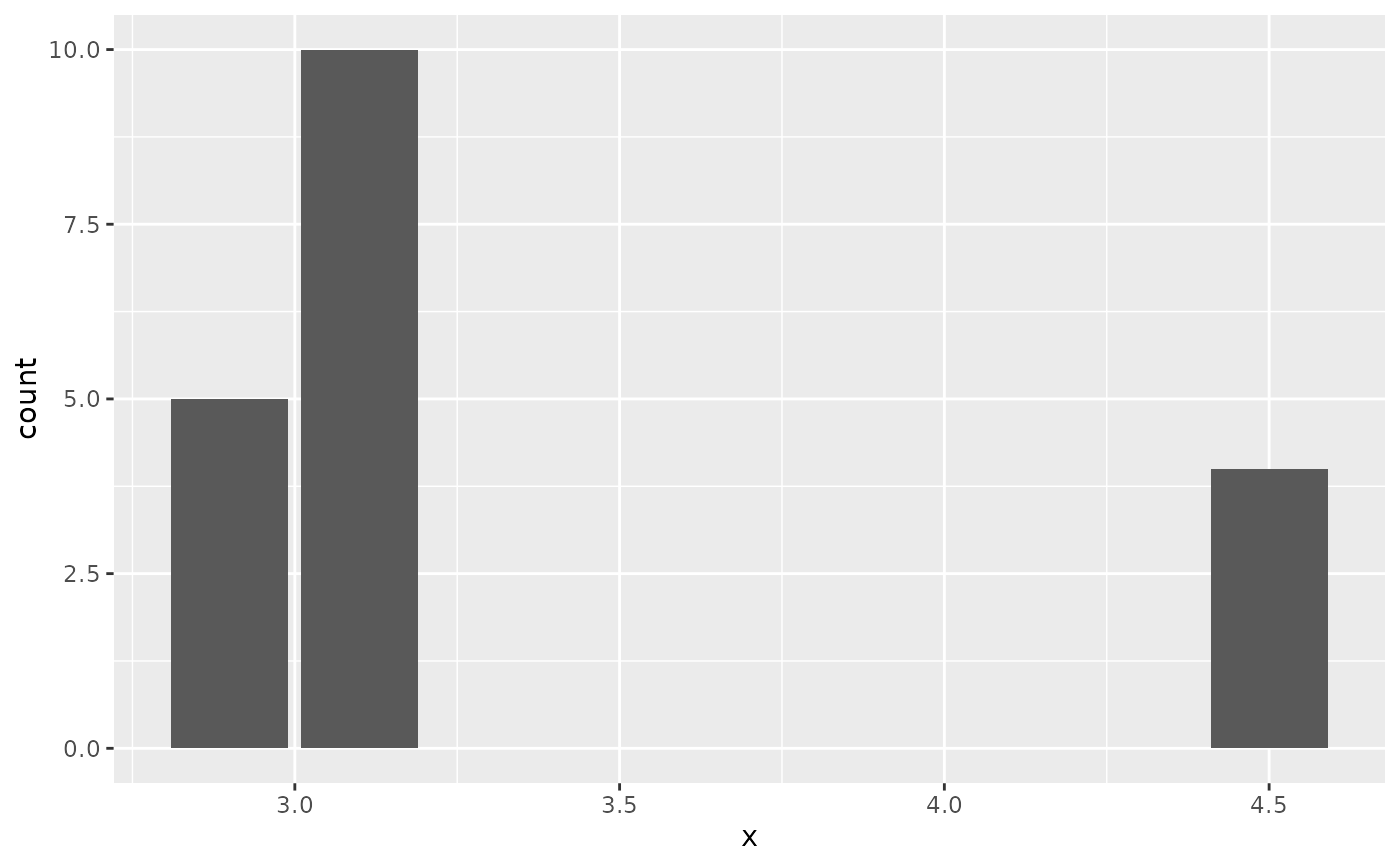# cf. a histogram of the same data
ggplot(df, aes(x)) + geom_histogram(binwidth = 0.5)